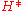xNormal heightEncyclopedia
Normal heights are heights above sea level, one of several types of height which are all computed slightly differently. Alternatives are: orthometric height
Orthometric height
The orthometric height is the distance H along a line of force from a given point P at the physical surface of an object to the geoid.Orthometric heights are what are usually used in the US for ordinary engineering work. Values for measured points can be obtained from the National Geodetic Survey...

s and dynamic height
Dynamic height
Dynamic height is a way of specifying the height of a point above a reference, as opposed to orthometric height or normal height.Dynamic height is constant if one follows the same gravity potential as they move from place to place. Because of variations in gravity, surfaces having a constant...

s.

The normal heightof a point is computed from geopotential numbers
Geopotential height
Geopotential height is a vertical coordinate referenced to Earth's mean sea level — an adjustment to geometric height using the variation of gravity with latitude and elevation. Thus it can be considered a "gravity-adjusted height"...

by dividing the point's geopotential number, i.e. its geopotential difference with that of sea level, by the average, normal gravity computed along the plumbline of the point. (More precisely, along the ellipsoidal normal, averaging over the height range from 0 — the ellipsoid — to; the procedure is thus recursive.

Normal heights are thus dependent upon the reference ellipsoid chosen. The Soviet Union and many other Eastern European countries have chosen a height system based on normal heights, determined by geodetic precise levelling. Normal gravity values are easy to compute and "hypothesis-free", i.e., one does not have to know, as one would for computing orthometric heights, the density of the Earth's crust around the plumbline.

Normal heights figure prominently in the theory of the Earth's gravity field developed by the school of M.S. Molodenskii. The reference surface that normal heights are measured from is called the quasi-geoid, a representation of "mean sea level" similar to the geoid
Geoid
The geoid is that equipotential surface which would coincide exactly with the mean ocean surface of the Earth, if the oceans were in equilibrium, at rest , and extended through the continents . According to C.F...

and close to it, but lacking the physical interpretation of an equipotential surface.